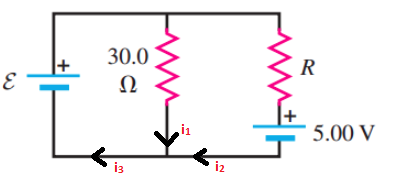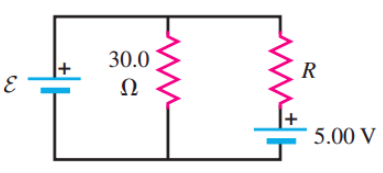# Problem: The batteries shown in the circuit in (Figure 1) have negligibly small internal resistances. Assume that &amp; 8.00 negligibly small internal resistances. Assume that-8.00 V and R = 21.9 Ω.1.Find the current through the 30.0 Ω resistor.Express your answer in amperes to three significant figures2.Find the current through the 21.9 Ω resistor.Express your answer in amperes to three significant figures3.Find the current through the 8.00 V battery.Express your answer in amperes to three significant figures

###### FREE Expert Solution(1)

Applying Kirchoff's loop rule to the left loop:

ε - (30.0)i1 = 0

i1 = ε/30.0 = 8.00/30.0 = 0.267 A

85% (223 ratings)###### Problem Details

The batteries shown in the circuit in (Figure 1) have negligibly small internal resistances. Assume that & 8.00 negligibly small internal resistances. Assume that-8.00 V and R = 21.9 Ω.1.

Find the current through the 30.0 Ω resistor.

2.

Find the current through the 21.9 Ω resistor.

3.

Find the current through the 8.00 V battery.